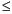Functions and CALL Routines

# RANBIN Function

Returns a random variate from a binomial distribution.
 Category: Random Number Tip: If you want to change the seed value during execution, you must use the CALL RANBIN routine instead of the RANBIN function.

## Syntax

 RANBIN(seed,n,p)

### Arguments

seed

is a numeric constant, variable, or expression with an integer value. If seed0, the time of day is used to initialize the seed stream.

n

is a numeric constant, variable, or expression with an integer value that specifies the number of independent Bernoulli trials parameter.

 Range: n> 0
p

is a numeric constant, variable, or expression that specifies the probability of success.

 Range: 0 < p < 1

The RANBIN function returns a variate that is generated from a binomial distribution with mean np and variance np(1-p). If n50, np5, or n(1-p)5, an inverse transform method applied to a RANUNI uniform variate is used. If n > 50, np > 5, and n(1-p) > 5, the normal approximation to the binomial distribution is used. In that case, the Box-Muller transformation of RANUNI uniform variates is used.

For a discussion about seeds and streams of data, as well as examples of using the random-number functions, see Generating Multiple Variables from One Seed in Random-Number Functions.

The CALL RANBIN routine, an alternative to the RANBIN function, gives greater control of the seed and random number streams.

 Functions and CALL routines:Previous Page | Next Page | Top of Page